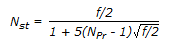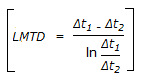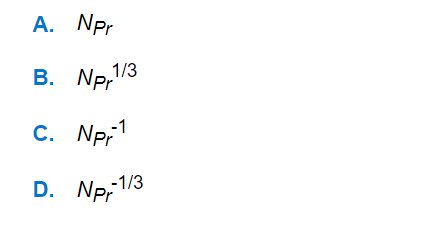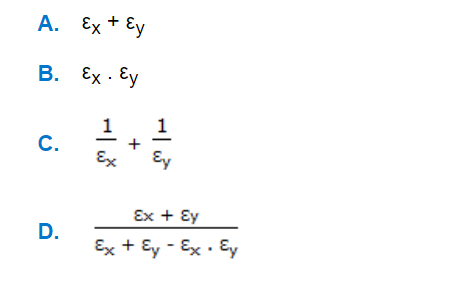# E-PolyLearning

 1. Reynold's analogy states thata. A b. B c. C d. D

 2. The following equation, corresponds to __________ analogy.a. Von-Karmann b. Reynolds c. Colburn d. Prandtl
 3. Out of the following four assumptions used in the derivation of the equation for LMTD given by below equation, which one is subject to the largest deviation in practice ?a. Constant overall heat transfer co-efficient. b. Constant rate of fluid flow. c. Constant specific heat. d. No partial phase change in the system.
 4. For flow over a flat plate, the ratio of thermal boundary layer thickness, 'xt' and hydrodynamic boundary layer thickness 'x' is equal to (where, NPr = Prandtl number)a. A b. B c. C d. D
 5. The interchange factor for radiation heat transfer from surface 'x' to surface 'y' in case of an infinite parallel planes with emis-sivities εx & εy is given bya. A b. B c. C d. D